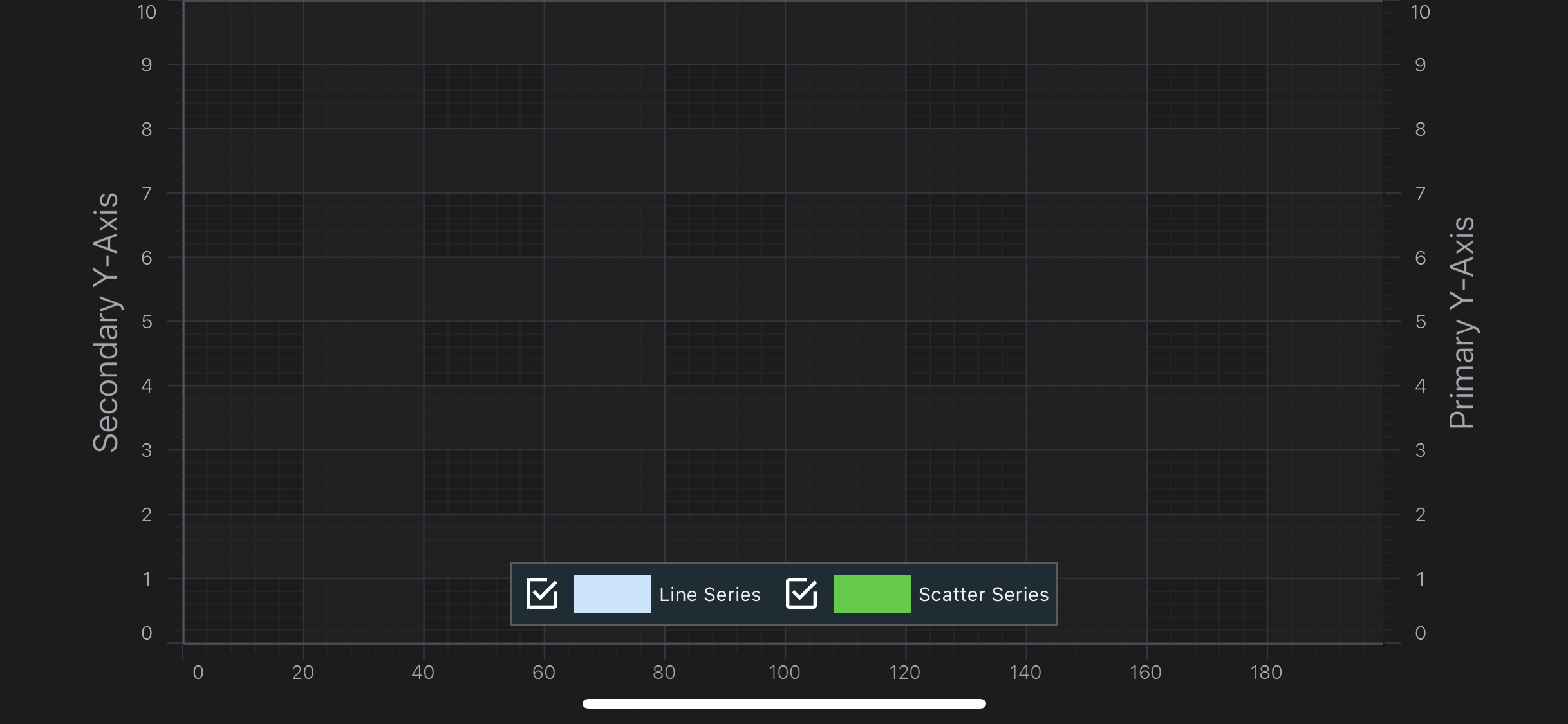iOS & macOS Charting Documentation - SciChart iOS & macOS Charts SDK v4.x

# SciChart iOS Tutorial - Multiple Axis

So far, in our series of tutorials, all the charts have had one `X-Axis` and one `Y-Axis`.

SciChart supports unlimited, multiple X, and Y Axis on the left, right, bottom and top of the chart. You can change Axis alignment, rotate charts, mix axis (have both XAxis/YAxis on the left), horizontally or vertically stack axes. The possibilities are literally endless!

In this tutorial, we are going to:

• add a second `Y-Axis` to the chart.
• show how to register annotations and line series on the second axis.
• ensure Axis drag behaviors work on both axis.

## Getting Started

This tutorial is suitable for Objective-C, Swift and C# with Xamarin.iOS.

NOTE: Source code for this tutorial can be found at our Github Repository:

First of all, make sure, you’ve went through the previous the tutorials:

And have at least basic understanding of how to use SciChart.

The procedure to add a second axis to a `SCIChartSurface` is pretty much the same as with one axis with one difference. You must assign a unique string ID to all axes if there is more than one.

To see the axis to appear to the either side of a chart, you set `SCIAxisAlignment` to e.g.:

• `SCIAxisAlignment.SCIAxisAlignment_Left`
• `SCIAxisAlignment.SCIAxisAlignment_Right`
• etc…

Let’s add another axis and align it to the left side of the chart (assuming the original one is placed to the right).

To have a different scale on the secondary axis, we are going to enlarge its VisibleRange by 40% setting a `ISCIAxisCore.growBy` value:

// Create another numeric axis, right-aligned SCINumericAxis *yAxisRight = [SCINumericAxis new]; yAxisRight.axisTitle = @“Primary Y-Axis”; yAxisRight.axisId = @“Primary Y-Axis”; yAxisRight.axisAlignment = SCIAxisAlignment_Right; // Create another numeric axis, left-aligned SCINumericAxis *yAxisLeft = [SCINumericAxis new]; yAxisLeft.axisTitle = @“Secondary Y-Axis”; yAxisLeft.axisId = @“Secondary Y-Axis”; yAxisLeft.axisAlignment = SCIAxisAlignment_Left; yAxisLeft.growBy = [[SCIDoubleRange alloc] initWithMin:0.2 max:0.2]; // … [self.surface.xAxes add:[SCINumericAxis new]]; [self.surface.yAxes addAll:yAxisLeft, yAxisRight, nil];
// Create another numeric axis, right-aligned let yAxisRight = SCINumericAxis() yAxisRight.axisTitle = “Primary Y-Axis” yAxisRight.axisId = “Primary Y-Axis” yAxisRight.axisAlignment = .right // Create another numeric axis, left-aligned let yAxisLeft = SCINumericAxis() yAxisLeft.axisTitle = “Secondary Y-Axis” yAxisLeft.axisId = “Secondary Y-Axis” yAxisLeft.axisAlignment = .left yAxisLeft.growBy = SCIDoubleRange(min: 0.2, max: 0.2) // … self.surface.xAxes.add(items: SCINumericAxis()) self.surface.yAxes.add(items: yAxisRight, yAxisLeft)
// Create another numeric axis, right-aligned var yAxisRight = new SCINumericAxis(); yAxisRight.AxisTitle = “Primary Y-Axis”; yAxisRight.AxisId = “Primary Y-Axis”; yAxisRight.AxisAlignment = SCIAxisAlignment.Right; // Create another numeric axis, left-aligned var yAxisLeft = new SCINumericAxis(); yAxisLeft.AxisTitle = “Secondary Y-Axis”; yAxisLeft.AxisId = “Secondary Y-Axis”; yAxisLeft.AxisAlignment = SCIAxisAlignment.Left; yAxisLeft.GrowBy = new SCIDoubleRange(0.2, 0.2); // … Surface.XAxes.Add(new SCINumericAxis()); Surface.YAxes.Add(yAxisLeft); Surface.YAxes.Add(yAxisRight);

Now we can see the second axis in our application:NOTE: Annotations and RenderableSeries don’t get rendered now

## Registering RenderableSeries on the Second Y-Axis

If there are several Y or X axes, you need to register other chart parts, like RenderableSeries and Annotations, on a particular axis to be measured against its scale.

From the tutorial, we are going to attach one series to the right axis and the other to the left axis, passing corresponding IDs to the RenderableSeries:

SCIFastLineRenderableSeries *lineSeries = [SCIFastLineRenderableSeries new]; lineSeries.yAxisId = @“Primary Y-Axis”; SCIXyScatterRenderableSeries *scatterSeries = [SCIXyScatterRenderableSeries new]; scatterSeries.yAxisId = @“Secondary Y-Axis”;
let lineSeries = SCIFastLineRenderableSeries() lineSeries.yAxisId = “Primary Y-Axis” let scatterSeries = SCIXyScatterRenderableSeries() scatterSeries.yAxisId = “Secondary Y-Axis”
var lineSeries = new SCIFastLineRenderableSeries { DataSeries = lineDataSeries, YAxisId = “Primary Y-Axis” }; var scatterSeries = new SCIXyScatterRenderableSeries { YAxisId = “Secondary Y-Axis”, DataSeries = scatterDataSeries, PointMarker = new SCIEllipsePointMarker { FillStyle = new SCISolidBrushStyle(0xFF32CD32), Size = new CGSize(10, 10) } };

Which results in the following:

## Registering Annotations on the Second Y-Axis

Annotations also need to be registered on a certain axis in a multi-axis scenario. So we are going to alternate the axis IDs to annotations in on our chart for the left or right axes in the following way:

SCITextAnnotation *label = [SCITextAnnotation new]; label.yAxisId = x % 200 == 0 ? @“Primary Y-Axis” : @“Secondary Y-Axis”;
let label = SCITextAnnotation() label.yAxisId = x % 200 == 0 ? “Primary Y-Axis” : “Secondary Y-Axis”
var label = new SCITextAnnotation(); label.YAxisId = x % 200 == 0 ? “Primary Y-Axis” : “Secondary Y-Axis”;

If you now add `SCIYAxisDragModifier` you can see which series and annotation are registered on which `Y-Axis`: